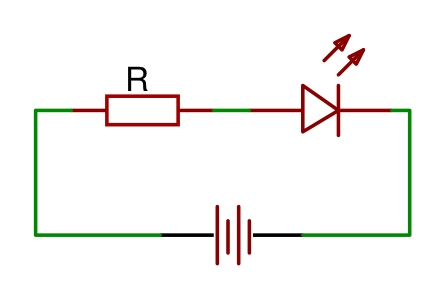# LED resistor calculator

The LED is used in nearly every electronics project. However, LEDs have a maximum voltage and you might damage your LEDs if you surpass it. It is, therefore, very important that you use a resistor to limit the supply voltage.

### Formula

The resistance of the resistor can be calculated with the following formula:V source is the source voltage and is measured in volt (V)
V LED is the LED voltage and is measured in volt (V)
I LED is the LED current and is measured in ampere (A)
R is the symbol for resistance and is measured in ohm (Ω).### Calculator

Enter three values to calculate the remaining one.